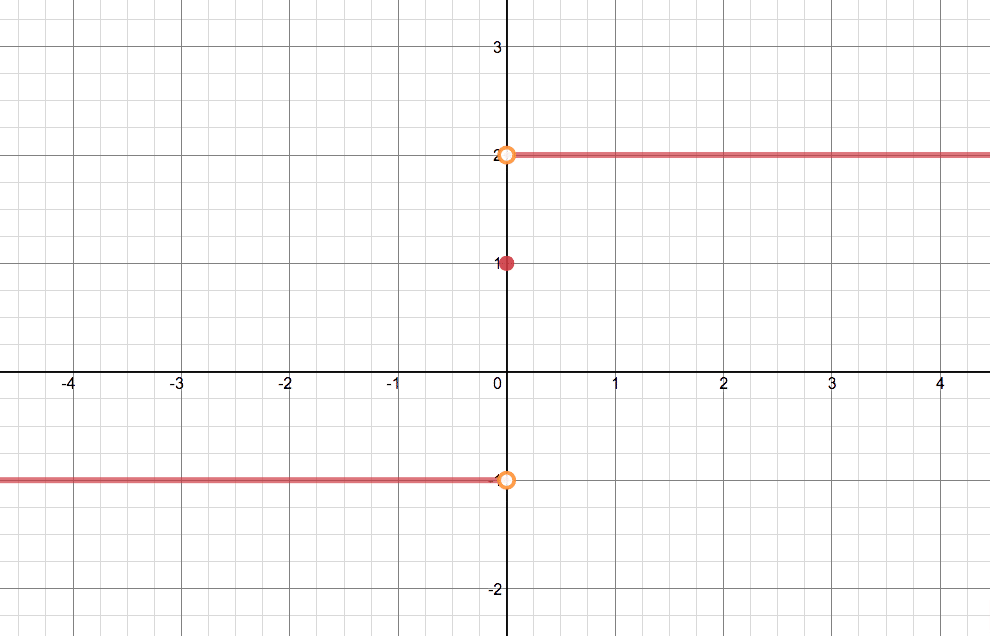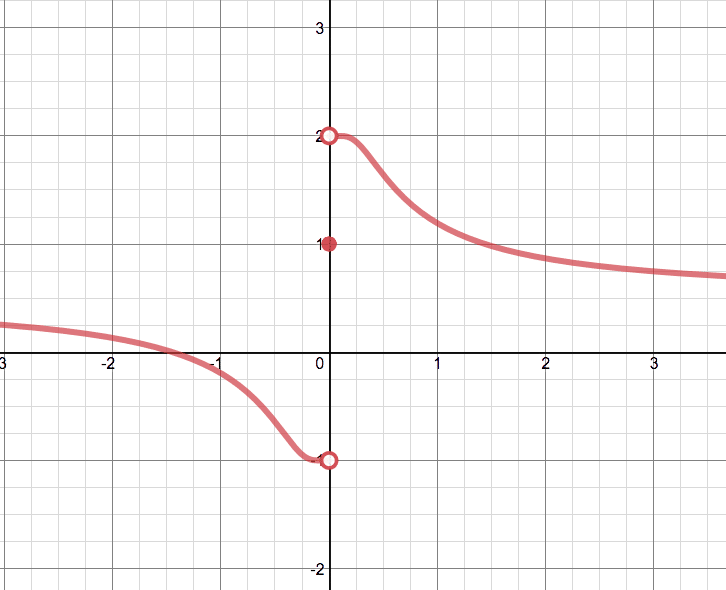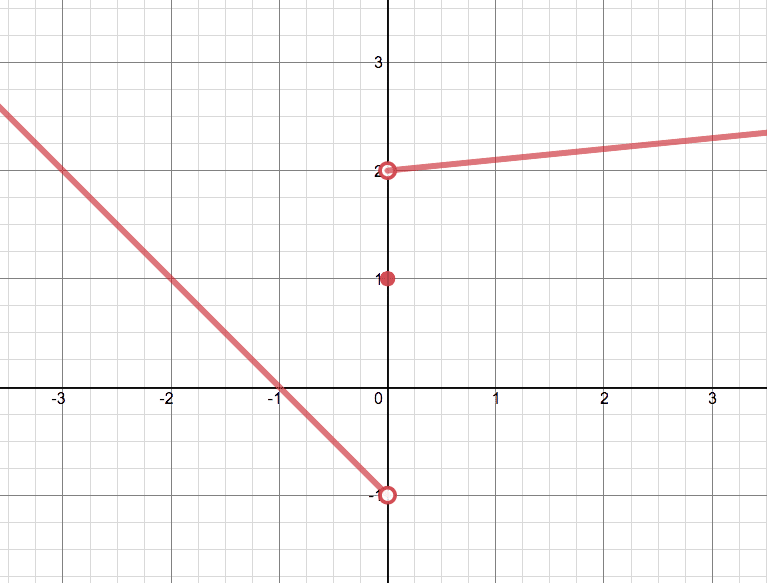# Sketch The Graph Of An Example Of A Function F That Satisfies

We thoroughly check each answer to a question to provide you with the most correct answers. Found a mistake? Let us know about it through the REPORT button at the bottom of the page.

Sketch the graph of an example of a function f that satisfies all of the given conditions. lim x→0- f(x)=-1, lim x→0+ f(x)=2, f(0)=1

Contents

There are many possible graphs that satisfy the given conditions, I will give three examples.

Note that the Limit from the left side must be -1

The functional value, represented by a filled dot is 1

And the Limit from the Right side is 2## Result

Note that the Limit from the left side must be -1

The functional value, represented by a filled dot is 1

And the Limit from the Right side is 2